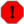## Name

ST_Length — Returns the 2D length of the geometry if it is a LineString or MultiLineString. geometry are in units of spatial reference and geography are in meters (default spheroid)

## Synopsis

`float ST_Length(`geometry a_2dlinestring`)`;

`float ST_Length(`geography geog, boolean use_spheroid=true`)`;

## Description

For geometry: Returns the 2D Cartesian length of the geometry if it is a LineString, MultiLineString, ST_Curve, ST_MultiCurve. 0 is returned for areal geometries. For areal geometries use ST_Perimeter. For geometry types, units for length measures are specified by the spatial reference system of the geometry.

For geography types, the calculations are performed using the inverse geodesic problem, where length units are in meters. If PostGIS is compiled with PROJ version 4.8.0 or later, the spheroid is specified by the SRID, otherwise it is exclusive to WGS84. If `use_spheroid=false`, then calculations will approximate a sphere instead of a spheroid.

Currently for geometry this is an alias for ST_Length2D, but this may change to support higher dimensions.Changed: 2.0.0 Breaking change -- in prior versions applying this to a MULTI/POLYGON of type geography would give you the perimeter of the POLYGON/MULTIPOLYGON. In 2.0.0 this was changed to return 0 to be in line with geometry behavior. Please use ST_Perimeter if you want the perimeter of a polygonFor geography measurement defaults spheroid measurement. To use the faster less accurate sphere use ST_Length(gg,false);This method implements the OpenGIS Simple Features Implementation Specification for SQL 1.1. s2.1.5.1This method implements the SQL/MM specification. SQL-MM 3: 7.1.2, 9.3.4

Availability: 1.5.0 geography support was introduced in 1.5.This method is also provided by SFCGAL backend.

## Geometry Examples

Return length in feet for line string. Note this is in feet because EPSG:2249 is Massachusetts State Plane Feet

```SELECT ST_Length(ST_GeomFromText('LINESTRING(743238 2967416,743238 2967450,743265 2967450,
743265.625 2967416,743238 2967416)',2249));
st_length
---------
122.630744000095

--Transforming WGS 84 LineString to Massachusetts state plane meters
SELECT ST_Length(
ST_Transform(
ST_GeomFromEWKT('SRID=4326;LINESTRING(-72.1260 42.45, -72.1240 42.45666, -72.123 42.1546)'),
26986
)
);
st_length
---------
34309.4563576191
```

## Geography Examples

Return length of WGS 84 geography line

```			-- default calculation is using a sphere rather than spheroid
SELECT ST_Length(the_geog) As length_spheroid,  ST_Length(the_geog,false) As length_sphere
FROM (SELECT ST_GeographyFromText(
'SRID=4326;LINESTRING(-72.1260 42.45, -72.1240 42.45666, -72.123 42.1546)') As the_geog)
As foo;
length_spheroid  |  length_sphere
------------------+------------------
34310.5703627288 | 34346.2060960742
```